# Numerical Analysis of Concentrated Beam Solar Circular Receivers

Numerical Analysis of Concentrated Beam Solar Circular Receivers
School of Mech. Eng., Dr. Vishwanath Karad MIT World Peace University, Pune 411038, India

Corresponding Author Email:
Kaustubh.Kulkarni@mitwpu.edu.in
Page:
375-382
|
DOI:
https://doi.org/10.18280/ijht.400203
1 February 2022
|
Accepted:
23 February 2022
|
Published:
30 April 2022
| Citation

OPEN ACCESS

Abstract:

The solar central receiver is the most crucial part of a solar tower power plant (CSP). In this study, a computational fluid dynamics (CFD) framework was used for analysing four circular designs (with same surface area and mass flow rate of heat transfer fluid) of the central tower receiver. In this study, a computational fluid dynamics (CFD) framework was developed for analyzing four circular (stain steel) designs of the central tower receiver, namely, a circular constant tube diameter (CCD), a circular vertical constant tube diameter solar receiver (CUTD), a circular variable tube diameter (CVTD) receiver and a leaf type circular solar receiver (LTSR). This analysis studied the solar radiation heat transfer efficiency, temperature distribution, and fluid outlet temperature; pressure and velocity distributions for the designs using CFD. It was found that the LTSR design helped achieve a higher rise in temperature of the heat transfer fluid (HTF) when the mass flow rate was in the range of 0.1 to 0.2 liter per minute. The LSTR model of circular receiver was more efficient in heat transfer circular receiver designs compared with other circular designs for same surface area and strength of solar beam irradiations.

Keywords:

1. Introduction

For millions of years, solar energy has been a natural vital force for all geomorphological changes on Earth . Conventional energy sources (coal, oil, and natural gas) have higher power densities. If conventional energy sources are utilized, the toxic gases emitted into the atmosphere pollute the environment . In a CSP system, a specific arrangement of mirrors (heliostats) reflects and concentrates solar radiation on a receiver atop a tower. This concentrated solar beam is then utilised as a heating source to heat a heat transfer fluid (HTF) circulating in the piping of the central solar receiver. Solar energy is a clean and alternative energy source  that needs to be explored. Because it is a dilute source of energy, it may not be directly utilised to generate power , but if this dilute source is utilised as input to systems, a concentrated high-energy solar beam could then be utilised for domestic, industrial, or power generation .

2. Literature Review

Concentrated solar radiation is a high-temperature, high-energy source . This radiation is utilised as thermal energy when it is directed at a receiver or concentrating device . Several authors have looked into incorporating solar thermal power into high-temperature production processes, using Computational Fluid Dynamics (CFD) to analyze high-temperature solar devices for improving prototype designs and the high-temperature process' performance .

Intense sunlight irradiates the tubes of the receiver in a concentrated solar thermal power plant, converting solar power into heat. The heat flux density on the receiver surface may be approximately 2.5 MW/m2. The heat flux density distribution on the receiver surface is determined by the aiming method . This heat flux is transferred from the receiver tube walls to the heat transfer fluid (HTF), which is circulated in the receiver (heat exchanger) system .

A greater HTF temperature is achieved with an increased receiver concentrated solar beam temperature . Solar receivers are heat exchangers that transfer solar energy to heat energy through the use of water. Then the fluid transfers the thermal energy to steam that will then be passed through a turbine to generate electricity . The amount of solar irradiance reflected by the heliostats on the receiver determines the amount of heat input to the receiver . An analytical framework that could be employed for "n" no. of mirrors is utilised for selecting a tiny central receiver system, and an empirical investigation of temperature readings of working fluid in a spiral receiver was performed for variable heat flux and mass flow rate .

## 1.png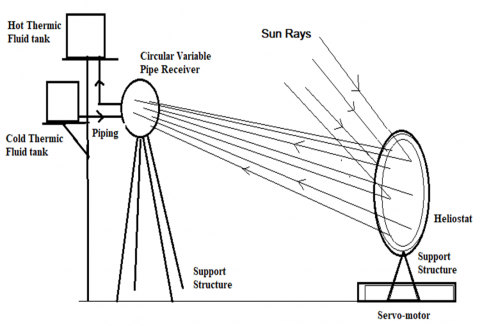Figure 1. CST layout with a receiver, heliostat, support structure system, etc.

The CFD results for a circular solar receiver are compared with a conventional solar receiver for the pressure drop and heat transfer coefficient . They found that the central portion gains more heat as compared to the peripheral tubes. A CFD model was developed to calculate the temperature profile in the wall of absorber tubes using steam as HTF . They found that the thermal stress in the absorber tubes is higher when the fluid is steam. Thus stainless steel tube needs to be used for the receiver. An analytical model was presented in the study  to evaluate the outlet temperature of the spiral receiver. They reported a maximum temperature of about 92℃ with a mass flow rate of 0.0016 Kg/sec at the solar noon in their experiments.

## 2.pngFigure 2. Temperature distribution of a solar beam concentrated on the receiver surface

3.1 Numerical modeling

Figure 6 shown a leaf type circular (LSTR) solar receiver. The receiver was designed such that the HTF enters the receiver from the bottom and leaves the receiver from the top, as indicated by points 1 and 2 in Figure 6, respectively. The tubes are inclined so that the advantage of siphon effect on the HTF can be utilized to move the hot HTF into upper levels of the receiver. The headers diameters were designed decreasing from the entry to the exit. A hot fluid collecting chamber was placed at the top of the LSTR as shown in Figure 6. All the models (Figures 3 to 6) were design such that they had the same surface area, so that they are exposed to an equal solar beam radiation under this analysis. The parameters used during the design of the four circular models of receivers are in Table 1.

## 3.png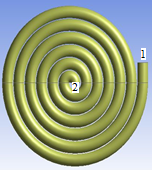Figure 3. Circular constant tube diameter (CCR) solar receiver. HTF enter at inlet (1) and leaves from outlet (2)

## 4.png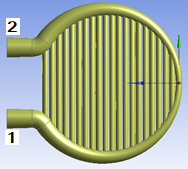Figure 4. Circular constant diameter, vertical tube (CUTD) solar receiver. HTF enter at inlet (1) and leaves from outlet (2)

## 5.pngFigure 5. Circular variable tube diameter (CVTD) solar receiver. HTF enter at inlet (1) and leaves from outlet (2)

## 6.png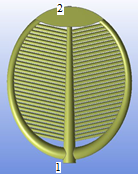Figure 6. Circular leaf type (LSTR) solar receiver. HTF enter at inlet (1) and leaves from outlet (2)

Table 1. Design parameters for circular receivers

 No Parameters 1 area of the receiver (m2) 0.6 2 tube thickness (mm) 1 3 mass flow rate (LPM) 0.1 to 0.5 4 heat flux (w/m2) 1000 to 3000 5 material of receiver Stainless Steel

3.2 Governing equations

In the numerical analysis, all the modelled solar receivers follow the following equations for continuity, momentum, and energy. (1) to (3) are the continuity equation, momentum, and energy equations, respectively.

$\frac{\partial u_{i}}{\partial x_{i}}=0$      (1)

$\frac{\partial u_{i} u_{j}}{\partial x_{i}}=-\frac{1}{\rho} \frac{\partial p}{\partial x_{i}}+\frac{\partial}{\partial x_{i}}\left(\left(v+v_{t}\right)\left(\frac{\partial u_{i}}{\partial x_{j}}+\frac{\partial u_{j}}{\partial x_{i}}\right)\right)$      (2)

$\frac{\partial u_{i} T}{\partial x_{i}}=\rho \frac{\partial}{\partial x_{i}}\left(\left(\frac{v}{P_{r}}+\frac{v_{t}}{P_{r}}\right) \frac{\partial T}{\partial x_{i}}\right)$      (3)

The domain definitions and boundary conditions for numerical simulations are as shown in Table 2. The SIMPLE algorithm was used with a finite-volume formulation to solve for convergence in the simulation. A standard scheme was used for the pressure term, and the first-order upwind scheme was adopted for the governing equations. Ansys® 2016 was used in the simulation of all the models with the same boundary conditions, mass flow rate, and irradiance flux on the surface of the receivers. The output from the software was recorded and analysed. The other settings used in the software during simulation are in Table 3.

Table 2. Boundary conditions settings

 Boundary Inlet Mass flow-Inlet Outlet pressure-Outlet Initial pressure (gauge) zero Pascal Inlet Temperature 300 K

3.3 Analysis procedure

Each of the circular receiver models was set for convergence. The domain definitions and boundary conditions for numerical simulations with the SIMPLE algorithm were used with a finite-volume formulation to solve for convergence in the simulation. A standard scheme was used for the pressure term, and the second-order upwind scheme was adopted for the governing equations. A grid independence test was conducted by varying the mesh size for the models. The output from the software was recorded and analyzed.

Table 3. Settings for ANSYS simulation

 Parameter Setting Working fluid Water Pipe material Stainless Steel (SS) Model used Energy Pressure Velocity    Coupling Scheme SIMPLE Spatial Gradient Green Gauss Cell Based Initialization Standard Computed for All zones

3.4 Mathematical modelling

The central receiver absorbs the sun's energy concentrated on it and transmits the heat absorbed to the working fluid (HTF). Tubes with excellent thermal conductivity and durability are used for high effectiveness and thermal efficiency. The availability of heat flux is high in the central inner region and low in the outer region (refer to Figure 2). The heat flow zone with the highest heat flux is in the centre of the receiver, whereas the heat flux zone with the lowest heat flux is on the outside. As a result, utilising a circular design for the receiver and lead tube was investigated over the conventional straight tube design. Therefore, the mathematical modelling of a receiver is explained as below.

The receiver tube's length of the available focus area is represented as,

$L=\pi * \frac{D_{0}+D_{1}}{2}$      (4)

Eq. (4) was multiplied by $\pi$ to obtain L in the circular case.

For the accessible mirror area, the theoretical heat rate on the circular receiver can be given as,

$Q=I_{B} \gamma_{b} \rho A_{m}$      (5)

The beam radiation are obtained using:

$\mathrm{I}_{B}=I_{G}-I_{D}$      (6)

The energy balance for a receiver can be written as follows:

$Q_{t}=Q_{a}+Q_{c}+Q_{\gamma}$      (7)

For the SS receiver, the density considered was 9078 kg/m3. The Cp assumed was 481 J/kg-K with thermal conductivity of 487 W/m-K. The absorbency fraction that was used in the anlysis software was 0.8, so the reflectivity was 0.2. That is, of the total in Eq. (7), (absorptivity) was 80% due to the material copper and (reflectivity) was 20%. Heat transfer coefficient due to convective losses.

$h=5.7+3.8 \mathrm{~V}$       (8)

The temperature of the working fluid at the outlet was determined using:

$\frac{I_{b} \gamma_{b} \rho \alpha A_{m}-h \cdot A_{0}\left(T_{w}-T_{a}\right)-\varepsilon \cdot A_{0} K_{b}\left(T_{\omega}^{4}-T_{a}^{4}\right)}{m c_{p}}$$+T_{i}=T_{0}$      (9)

If Q is the solar flux input/solar irradiation, then the heat absorbed by HTF was obtained (the product of the mass flow rate of HTF in kg/s, the specific heat capacity of water in J/kg.K, and temperature gain). The efficiency (ƞ) was the ratio of heat absorbed by HTF to the solar flux input.

4. Results and Discussions

The effectiveness of the all modelled circular solar receivers was evaluated using temperature, pressure, and velocity measures. The CFD output for temperature, pressure, and velocity for the modelled circulate constant tube diameter (CCR) solar receiver is presented in Figures 7 to 9 respectively. It was observed from Figure 7 that the HTF temperature had a peak value at the exit zone of the header for the CCR model. A lower HTF temperature zone was observed at the entry of the CCR model. The central zone of the CCR showed a higher temperature level for the HTF. The pressure variation (refer to Figure 8) of the HTF for the CCR suggests that there is a lower pressure at the eye of the CCR model (as seen by the blue shade) while there is a comparatively higher pressure developed in the peripheral tubes of the CCR. This phenomenon occurs continuously, so the blue colour area is always at a reduced temperature distribution. The velocity in the CCR tubes is approximately constant for any given mass flow rate of the HTF.

## 7.png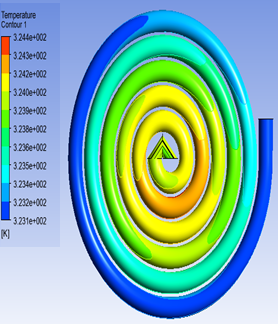Figure 7. Variation of HTF temperature in the CCR

## 8.pngFigure 8. Variation of HTF pressure in the CCR

## 9.png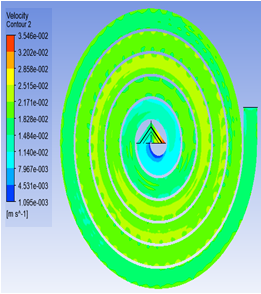Figure 9. Variation of HTF velocity in the CCR

## 10.pngFigure 10. Variation of HTF Temperature difference versus flow rate for CCR

## 11.png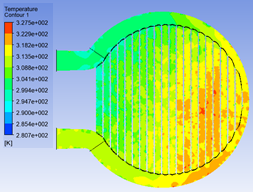Figure 11. Variation of HTF temperature in the CUTD

## 12.png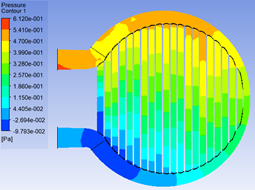Figure 12. Variation of HTF pressure in the CUTD

## 13.png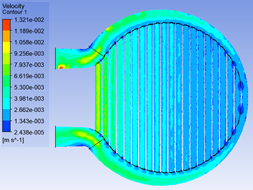Figure 13. Variation of HTF velocity in the CUTD

## 14.png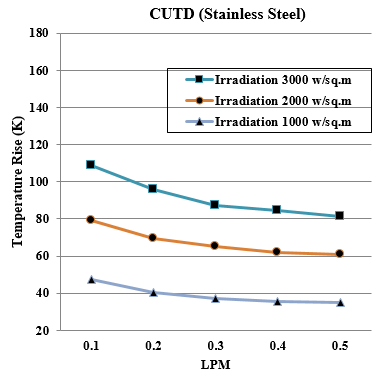Figure 14. Variation of HTF Temperature difference versus flow rate for CUTD

## 15.png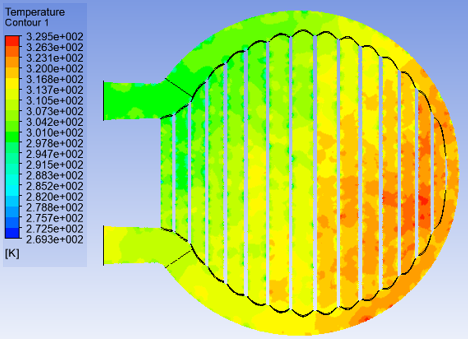Figure 15. Variation of HTF temperature in the CVTD

## 16.png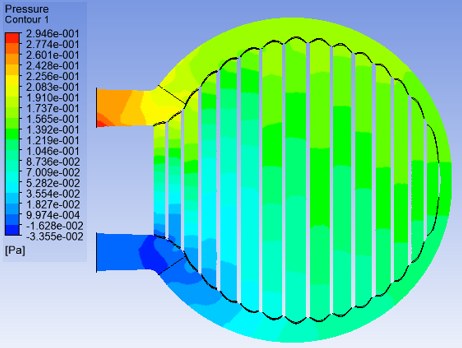Figure 16. Variation of HTF pressure in the CVTD

## 17.png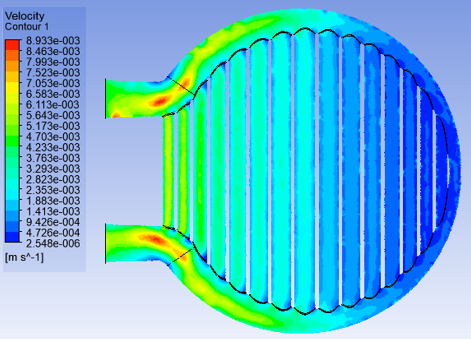Figure 17. Variation of HTF velocity in the CVTD

## 18.png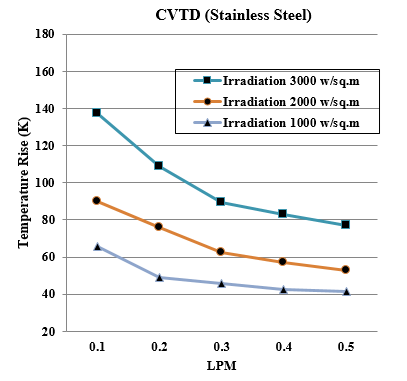Figure 18. Variation of HTF Temperature difference versus flow rate for CVTD

## 19.png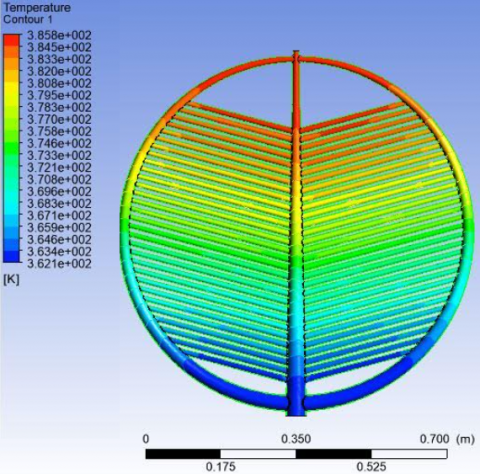Figure 19. Variation of HTF temperature in the LTSR

## 20.pngFigure 20. Variation of HTF pressure in the LTSR

## 21.png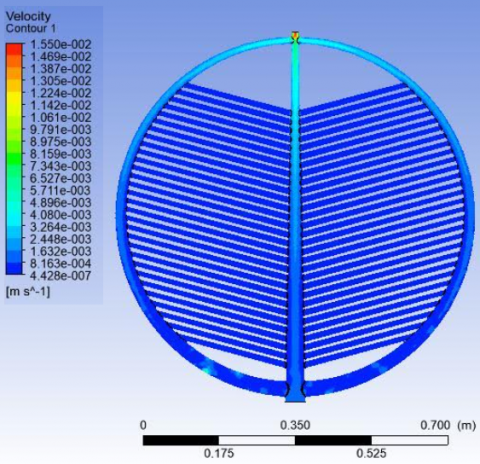Figure 21. Variation of HTF velocity in the LTSR

## 22.png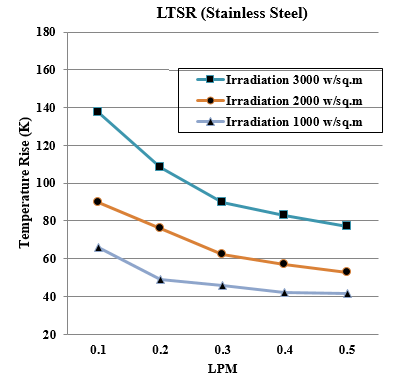Figure 22. Variation of HTF Temperature difference versus flow rate for LTSR

## 23.pngFigure 23. Variation of HTF temperature difference (To – Ti) versus HTF flow rate in LPM in receivers at 3000 W/m2 solar intensity

## 24.pngFigure 24. Variation of HTF temperature difference (To – Ti) versus HTF flow rate in LPM in receivers at 2000 W/m2 solar intensity

## 25.png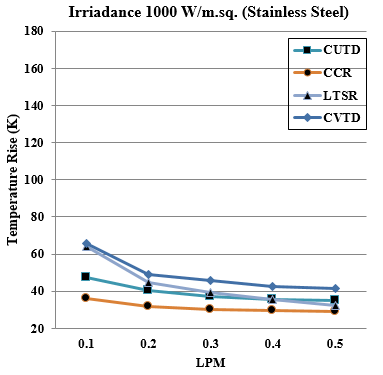Figure 25. Variation of HTF temperature difference (To – Ti) versus HTF flow rate in LPM in receivers at 1000 W/m2 solar intensity

The above discussion suggests that as the HTF moves from the lower irradiation zone (refer to Figure 2) from the periphery to the eye of the CCR, the HTF absorbs heat and moves to the eye of the CCR. The HTF density variation causes an incremental increase in the velocity of HTF and a smaller incremental decrease in the pressure of HTF in the CCR as the HTF moves from its periphery to its eye under the heat flux from solar irradiance. Figure 10 shows the plot of the temperature rise in the HTF versus the mass flow rate of HTF (water) in the CCR. It was found that for a lower mass flow rate of HTF in the CCR receiver, the temperature difference gained was higher as the HTF had more time to remain in the tubes of the CCR for a given incident irradiation heat flux in the analysis.

The results of the analysis for the circular vertical uniform tube diameter (CUTD) receiver model are presented in Figures 11 to 15. The tubes have a uniform tube diameter and are made of stainless steel. The HTF enters the CUTD receiver from the bottom header and exists in the CUTD from the top header. The vertical tubes receive solar irradiation of 1000 to 3000 W/m2 in the analysis under the boundary conditions set as per Table 2 and Table 3. From Figure 11, we observe that the gain in temperature of the HTF was away from the centre of the CUTD. The HTF took the shortest route (minimum resistance) after its entry point to move to the exit header.

The HTF velocity plot in Figure 13 for the CUTD receiver indicates a higher velocity in the first few vertical CUTD pipes. Consequently, there is lower pressure in the first few vertical CUTD pipes. This indicates that the volume of HTF in the further pipes of the CUTD receiver was smaller. Thus, in Figure 11, only the smaller quantity of HTF shows orange, indicating a higher temperature rise in further pipes of CUTD. Figure 15 shows the plot of the temperature rise of HTF against the mass flow rate in the CUTD receiver. It was observed from the graphs that a higher temperature difference (To – Ti) of the HTF was achieved for lower LPM of HTF through the CUTF receiver for reasons explained in the analysis of the CCR analysis.

The results of analysis for circular variable tube diameter (CVTD) receiver model are presented in Figures 13 to 16. The vertical tubes have variable tube diameter and are made of stainless-steel. The central vertical tube is of larger diameter and the diameters of tubes on the left and right of the center vary in decreasing order. The CVTD model has the same surface area of approximately 0.6 m2 as other models. The HTF enter the CVTD receiver from the bottom header and exists the CVTD from the top header. The CVTD tubes receive the solar irradiations of 1000 to 3000 W/m2 in the analysis under the boundary conditions set as per Table 2 and Table 3.

From Figure 15, we observe that the gain in temperature of the HTF was away from the center of the CVTD. The HTF took the shortest route (minimum resistance) after its center point to move to the exit header. The HTF velocity plot in Figure 17 for CVTD receiver indicates a higher velocity in the first few vertical CVTD tubes and medium velocity up to the central tubes of CVTD. The velocity of HTF in further tubes reduces. This suggests that the mass flow rate in the further tubes beyond the center of the CVTD is lower, thus a higher heated area is seen in Figure 15 for a given irradiation incident solar flux. Figure 18 is the plot of temperature rise of HTF against mass flow rate in the CVTD receiver for three different irradiation flux. It was observed from the graphs that a higher temperature difference (To – Ti) of the HTF was achieved for lower LPM of HTF though the CVTF receiver for reasons explain in the analysis of CUTD analysis.

Figure 19 is a plot of the temperature difference (To – Ti) of the HTF versus mass flow rate in litres per minute (LPM) using the LTSR model in the simulation. From Figure 19, it was found that, because of the shape of the LTSR model, for a lower mass flow rate of HTF, the temperature difference (To – Ti) is higher than the temperature difference (To – Ti) in the CCR and CUTD models. This suggests that the heat absorbed from the beam of solar radiation incident on the LTSR was utilised as HTF in a more effective and efficient way. The temperature gained by the HTF is higher as the HTF raises from the entry point (Point 1) to the exit point (Point 2) of the LTSR model. The pressure and velocity plots in Figures 20 and 21 are uniform, suggesting a uniform gain in temperature of the HTF across the LTSR model. The temperature gained (To – Ti) by various circular models of receivers versus the intensity of irradiations in the analysis is shown in Figures 22 to 25. For the three intensities considered in the analysis, the LTSR model has shown higher effectiveness in heat gained at lower mass flow rates of 3000 and 2000 w/m2 flux. The LTSR model shows a lower effectiveness in heat gained at lower mass flow rates for 1000 w/m2 flux because the flow resistance is greater, so the low mass flow rate does not add higher temperatures to the HTF. This is reflected again as the distribution of HTF in LTSR from its entry point to exit has generated equal pressure and the syphonic effect of the HTF as it is heated to rise to the exit point. The pressure and velocity plots in Figures 20 and 21 respectively also support this argument, as seen by the uniformity of its mapped output.

5. Conclusions

Four different central circular solar receivers of stainless steel having same surface area under varying mass flow rate with three different solar incident beams were analysed numerically. The temperature, velocity, and pressure distributions of the heat transfer fluid (water) for the four circular solar receiver modelled geometries were analysed using Ansys®2016. The solar beam temperature at the center of the beam which was higher was utlized to the maximum by the leaf type circular solar receiver model. It was found that the heat gained (or absorbed) by the heat transfer fluid in the leaf type circular solar receiver model was higher as compared to all other circular receiver models considered in the analysis.

Acknowledgements

We thank Dr. Vishwanath Karad MIT-World Peace University, Pune, for allowing us to use their CFD simulation software for this analysis.

Nomenclature
 Do outside diameter of the tube Di inside diameter of the tube L length of the tube n number of turns Q theoretical heat rate γb tilt factor ρ reflectivity of mirror Am mirror area (m2) IB solar beam radiation (w/m2) IG global radiation (w/m2) ID diffuse radiation (w/m2) Qt heat absorbed by receiver (W) Qa heat absorbed by working fluid (W) Qc convective losses (W) Qγ radiation loses (W) h heat transfer coefficient (w/m2 K) A0 the receiver absorber area (m2) Ta the Ambient temperature of air (K) ε the emissivity of tube material Tw mean temperature of tube surface (K) T0 the outlet temperature of working fluid (K) Ti the Inlet temperature of working fluid (K) m mass flow rate (Kg/sec) cp specific heat (kJ/Kg k) Abbreviation CSP Solar tower power plant CFD Computational fluid dynamics CCD Circular constant tube diameter CUTD Circular vertical constant tube diameter solar receiver CVTD Circular variable tube diameter LTSR Receiver and a leaf type circular solar receiver HTF Heat transfer fluid
References

 Verma, S.K., Sharma, K., Gupta, N.K., Soni, P., Upadhyay, N. (2020). Performance comparison of innovative spiral shaped solar collector design with conventional flat plate solar collector. Energy, 194: 116853. https://doi.org/10.1016/j.energy.2019.116853

 Fritsch, A., Uhlig, R., Marocco, L., Frantz, C., Flesch, R., Hoffschmidt, B. (2017). A comparison between Transient CFD & FEM simulations of solar central receiver tubes using molten salt and liquid metals. Solar Energy, 155: 259-266. https://doi.org/10.1016/j.solener.2017.06.022

 Boerema, N., Morrison, G., Taylor, R., Rosengarten, G. (2013). High temperature solar thermal central-receiver billboard design. Solar Energy, 97: 35-68. https://doi.org/10.1016/j.solener.2013.09.008

 Abuseada, M., Ozalp, N. (2020). Experimental and numerical study on a novel energy efficient variable aperture mechanism for a solar receiver. Solar Energy, 197: 396-410. https://doi.org/10.1016/j.solener.2020.01.020

 Ophoff, C., Abuseada, M., Ozalp, N., Moens, D. (2020). Systematic approach for design optimization of a 3 KW solar cavity receiver via Multiphysics analysis. Solar Energy, 206: 420-35. https://doi.org/10.1016/j.solener.2020.06.021

 Ali, B., Gilani, S.I.U.H., Al-Kayiem, H.H. (2016). Mathematical modeling of a developed central receiver based on evacuated solar tubes. MATEC Web of Conferences, 38: 02005. http://dx.doi.org/10.1051/matecconf/20163802005

 Liao, Z.R., Li, X., Xu, C., Chang, C., Wang, Z.F. (2014). Allowable flux density on a solar central receiver. Renewable Energy, 62: 747-753. https://doi.org/10.1016/j.renene.2013.08.044

 Schiel, W., Geyer, M., Carmona, R. (1987). The IEA/SSPS High Flux Experiment, Testing the Advanced Sodium Receiver at Heat Fluxes up to 2.5 Mw/M2. Springer Verlag.

 Vignarooban, K., Xu, X.H., Arvay, A., Hsu, K., Kannan, A.M. (2015). Heat transfer fluids for concentrating solar power systems – a review. Applied Energy, 146: 383-396. https://doi.org/10.1016/j.apenergy.2015.01.125

 Ozalp, N., JayaKrishna, D. (2010). CFD analysis on the influence of helical carving in a vortex flow solar reactor. Int J Hydrog Energy, 35: 6248e60. https://doi.org/10.1016/j.ijhydene.2010.03.100

 Jamel, M.S., Rahman, A.A., Shamsuddin, A.H. (2013). Advances in the integration of solar thermal energy with conventional and non-conventional power plants. Renewable and Sustainable Energy Reviews, 20: 71-81. https://doi.org/10.1016/j.rser.2012.10.027

 Khalaji Assadi, M., Gilani, S.I., Jun Yen, T.C., Abdul Karim, Z.A., Hj Baharudin, Z., Basrawi, M.F., Rahman, Md M., Mat Noor, M. (2016). DESIGN a solar hybrid air conditioning compressor system. MATEC Web of Conferences, 38: 02005.

 Zhu, G.D., Libby, C. (2017). Review and future perspective of central receiver design and performance. Abu Dhabi, United Arab Emirates. https://doi.org/10.1063/1.4984395

 Yu, Q., Fu, P., Yang, Y.H., et al. (2020). Modeling and parametric study of molten salt receiver of concentrating solar power tower plant. Energy, 200(7): 117505. https://doi.org/10.1016/j.energy.2020.117505

 Tawfik, M., Tonnellier, X., Sansom, C. (2018). Light source selection for a solar simulator for thermal applications: A review. Renewable and Sustainable Energy Reviews, 90: 802-813. https://doi.org/10.1016/j.rser.2018.03.059

 Roldán, M.I., Valenzuela, L., Zarza, E. (2013). Thermal analysis of solar receiver pipes with superheated steam. Applied Energy, 103: 73-84. https://doi.org/10.1016/j.apenergy.2012.10.021

 Digole, S.P., Karvinkoppa, M., Sanap, S. (2020). Thermal analysis of a spiral solar receiver for a small central receiver system: An experimental and numerical investigation. International Journal of Ambient Energy, 1-9. https://doi.org/10.1080/01430750.2020.1722744